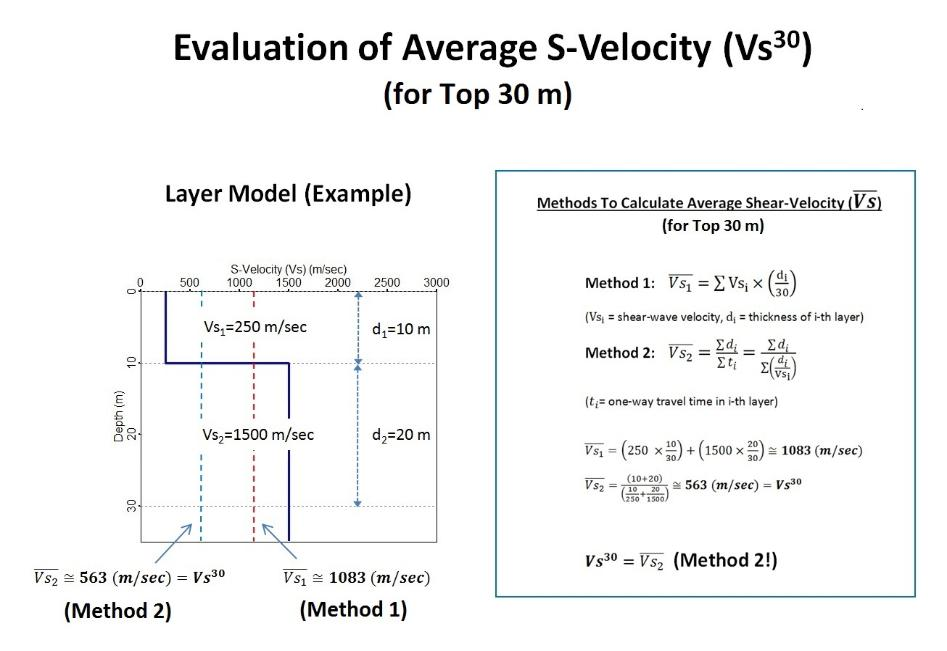# Calculating Vs30m

#### How to Calculate Average Vs and Vs30m

The average shear-wave velocity (Vs) for the upper 30-m depth is denoted as Vs30m (or Vs-30, Vs-30m, etc.). This is calculated from a Vs measurement presented in various formats.

Because of the gravitational influence, the property of ground materials is usually presented by, so-called, a "layered-earth-model" in which the earth's properties change only vertically and are represented by a collection of distinctive layers. Each layer is then considered a homogeneous material with the same seismic properties in S and P waves (Vs and Vp), and density. In addition, because of the more rapid property change at shallower depths, the thickness of each layer in a layered-earth-model tends to be smaller at the top, and increases with depth.

Vs measurements are also presented by a layered format as graphically illustrated below and also in some field examples of Vs30m. Calculation of the average Vs for a certain depth range (for example, top 30 m) can be accomplished in two different ways: (1) based on relative thickness-contribution of each layer (Method 1 in the illustration below), and (2) based on the definition of velocity ─ total thickness (∑di) divided by total travel time (∑ti) that is calculated by summation of thickness (di) divided by velocity (Vsi) of each layer (Method 2 in the illustration). Both methods can yield significantly different results for the same Vs profile as illustrated below by using a simple 2-layer Vs profile. Vs30m as defined in International Building Code (IBC 2000 and later editions) uses the second method (2):

Vs30m = ∑di / ∑ti = 30 / ∑(di/Vsi)